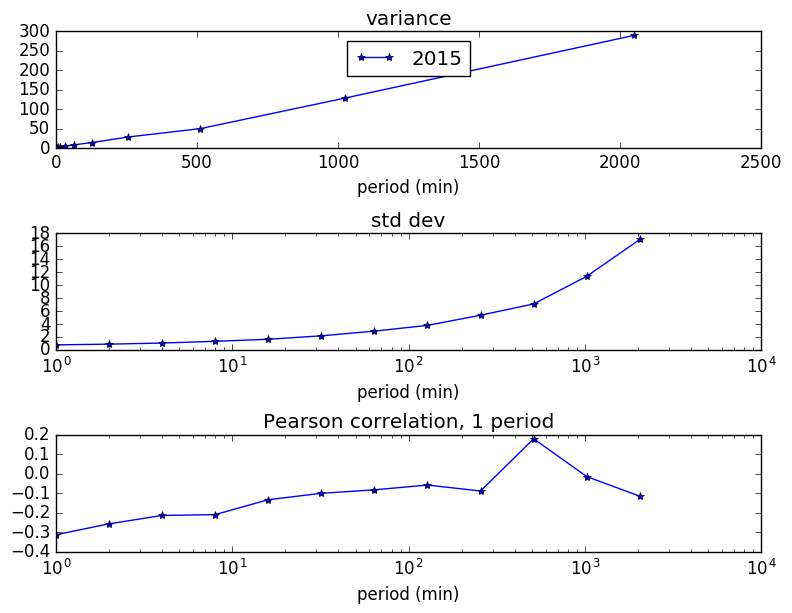This is part 2 of our Bitcoin analysis.  Following part 1 Do Bitcoin Prices Walk Randomly? we can apply some simple analysis on bitcoin trading profitability.

Recall that in the previous post, we learned that Bitcoin prices are slightly negatively correlated over various time periods. Thus we might ask the question, what if we followed a very simple strategy: Sell when prices rose in the prior period, and Buy when they fell. Our percentage profits would be roughly proportional to $$\sigma r / p$$ where $$\sigma$$ is the standard deviation of the price change, $$r$$ is the correlation coefficient, and $$p$$ is the current price. We analyzed the Bitcoin prices from the BTC-e exchange for 2015-2016. Here is the variation data (legend 2015 indicates aggregated data 2015-2016):Note that for short time periods (1 minute), there is more correlation in prices, but since the variance (standard deviation) is smaller, the profit on each trade will be smaller. This is where transaction costs come into play.

We now add two more things:

1. A threshold that a price change must exceed in order to make the trade
2. A transaction fee of 0.20%.  This is typical on Bitcoin exchanges.  Some might be less, depending on trading volume, and also Maker fees can be lower (where you place a limit order rather than execute against a current order)

You can see that we cannot quite get a positive profit on this admittedly simple trading strategy.  The correlation between prices changes is just not big enough to overcome the transaction fees.  You can see that as we raise the threshold, the profit per trade increases, but does not quite rise to the level of 0.20%, our transaction costs.